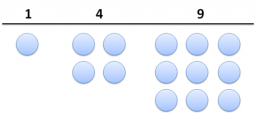Two expressions

When x = 3 and y = 5, by how much does the value of 3x2 – 2y exceed the value of 2x2 – 3y?

d =  14

Step-by-step explanation:Did you find an error or inaccuracy? Feel free to write us. Thank you!Tips to related online calculators
Do you have a linear equation or system of equations and looking for its solution? Or do you have a quadratic equation?